# Charged Particles Moving in a Magnetic Field Ranking Task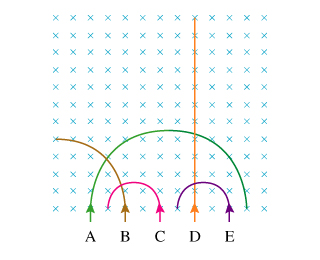Five equal-mass particles (A–E) enter a region of uniform magnetic field directed into the page. They follow the trajectories illustrated in the figure.

Which particle (if any) is neutral?

Which particle (if any) is negatively charged?

Rank the particles on the basis of their speed.

Concepts and reason

The concepts used in this problem are magnetic field, magnetic force and centripetal force. The ranking of the particles can be determined using the concept of magnetic field and magnetic force.

Fundamentals

Magnetic field

The region around a magnetic material or moving charge where the magnetic force acts is called the magnetic field. An electric charge is affected under the influence of magnetic field.

Centripetal Force:

The linear acceleration is the rate of change of linear velocity but the rate of change of tangential velocity is the centripetal acceleration. The centripetal acceleration makes an object to move in a curved path. The force acts in this motion is centripetal force

The expression for centripetal force is:

${F_{\rm{c}}} = \frac{{m{v^2}}}{r}$

Here, $m$ is the mass, ${a_{\rm{c}}}$ is the centripetal acceleration, $v$ is the velocity and $r$ is the radial distance.

Magnetic force:

The magnetic force is perpendicular to the magnetic field and the velocity of the charge. The magnitude of magnetic force is given by:

$\begin{array}{c}\\{{\vec F}_{{\rm{mag}}}} = q\left( {\vec v \times \vec B} \right)\\\\{F_{{\rm{mag}}}} = qvB\sin \theta \\\end{array}$

Here, $q$ is the charge, $v$ is the velocity, $B$ is the magnetic field and $\theta$ is the angle between velocity and the magnetic field.

(Part A)

All the particles are deflecting except particle D, it means that except D all other particles are charged particles. So, particle D is neutral.

(Part B)

The direction of magnetic field is into the plane. The particles B, C and E are moving in counterclockwise direction which is the direction according to Right Hand Thumb Rule for positive charge.

So, for negative charge, the direction should be clockwise. Particle A moves in clockwise direction.

(Part D)

For a single particle, the magnetic force is:

${F_{{\rm{mag}}}} = qvB\sin \theta$

Substitute ${90^{\rm{o}}}$ for $\theta$ .

$\begin{array}{c}\\{F_{{\rm{mag}}}} = qvB\sin {90^{\rm{o}}}\\\\ = qvB\\\end{array}$

The magnetic force should be balanced by centripetal force.

${F_{\rm{c}}} = \frac{{m{v^2}}}{r}$

$\begin{array}{c}\\{F_{{\rm{mag}}}} = {F_{\rm{c}}}\\\\qvB = \frac{{m{v^2}}}{r}\\\\v = \frac{{qBr}}{m}\\\end{array}$

The mass of all the particle are equal, but no information is given about the magnitude of charge, so the ranking cannot be determined.

Ans: Part A

The neutral particle is particle D.

Part B

The negatively charge particle is particle A.

Part D

The correct ranking of the speed of the particles cannot be determined.

The correct ranking cannot be determined.

particle d is neutralparticle a is negatively charged

This video explains it!! https://youtu.be/bvTb6dWLvpQ

#### Earn Coin

Coins can be redeemed for fabulous gifts.

Similar Homework Help Questions
• ### Charged Particles Moving in a Magnetic Field Ranking Task Five equal-mass particles (A?E) enter a region of...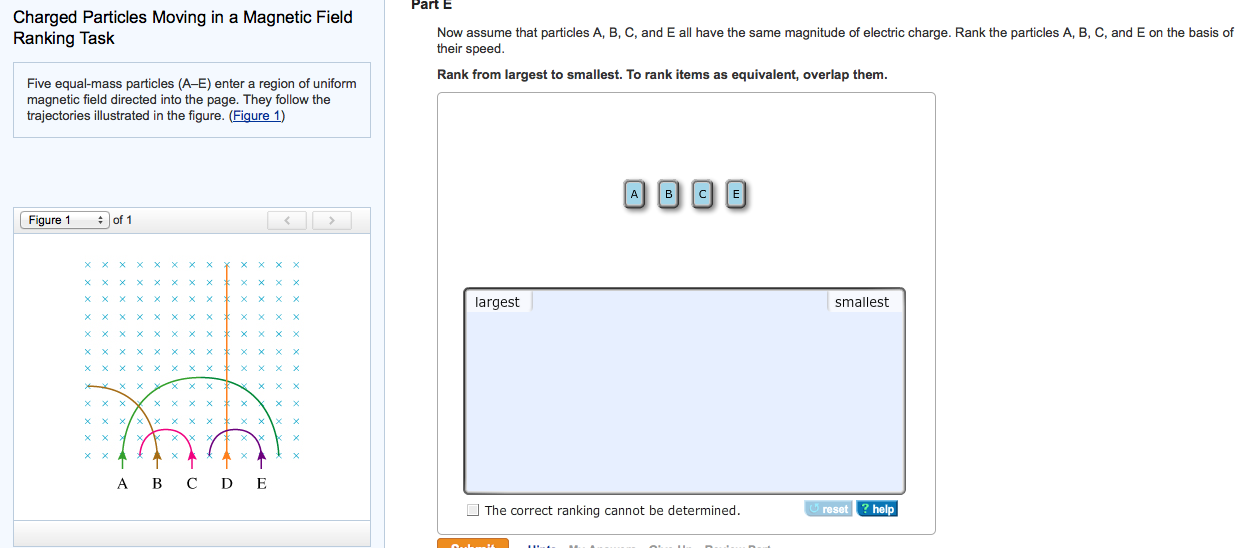Charged Particles Moving in a Magnetic Field Ranking Task Five equal-mass particles (A?E) enter a region of uniform magnetic field directed into the page. They follow the trajectories illustrated in the figure. (Figure 1)Now assume that particles A, B, C, and E all have the same magnitude of electric charge. Rank the particles A, B, C. and E on the basis of their speed. Rank from largest to smallest. To rank items as equivalent, overlap them.

• ### Five equal-mass particles (A-E) enter (with equal speed) into a region of uniform magnetic field directed...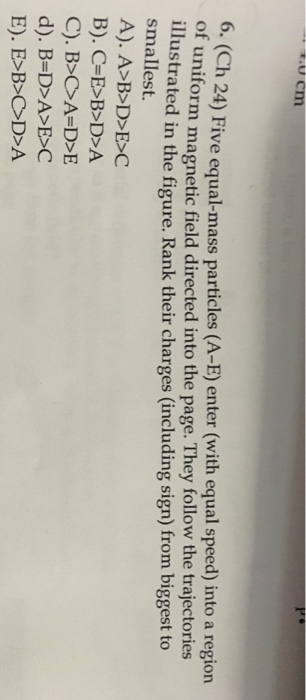Five equal-mass particles (A-E) enter (with equal speed) into a region of uniform magnetic field directed into the page. They follow the trajectories illustrated in the figure. Rank their charges (including sign) from biggest to smallest. A > B > D > E > C C = E > B > D > A B > C > A = D > E B = D > A > E > C E > B > C > D >...

• ### 10. Five equal-mass particles (A-E) enter a region of uniform B-field directed into the page and...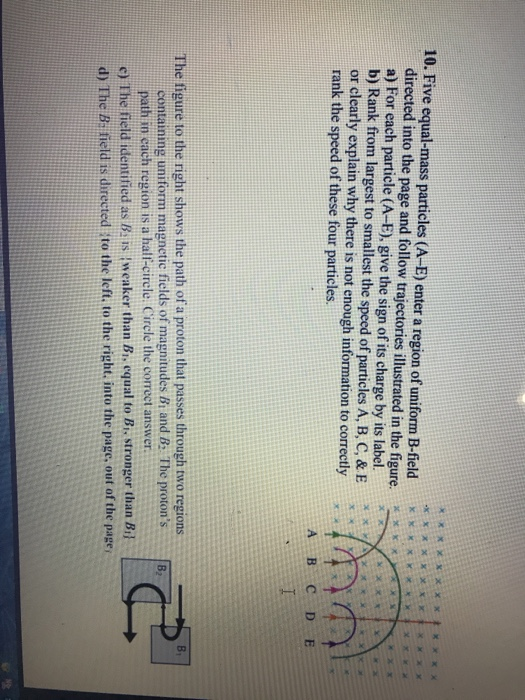10. Five equal-mass particles (A-E) enter a region of uniform B-field directed into the page and follow trajectories illustrated in the figure. a) For each particle (A-E), give the sign of its charge by its label. b) Rank from largest to smallest the speed of particles A, B, C, & E or clearly explain why there is not enough information to correctly rank the speed of these four particles, B C D E The figure to the right shows the...

• ### Two particles, alpha and beta, with the same speed enter a region with a uniform magnetic...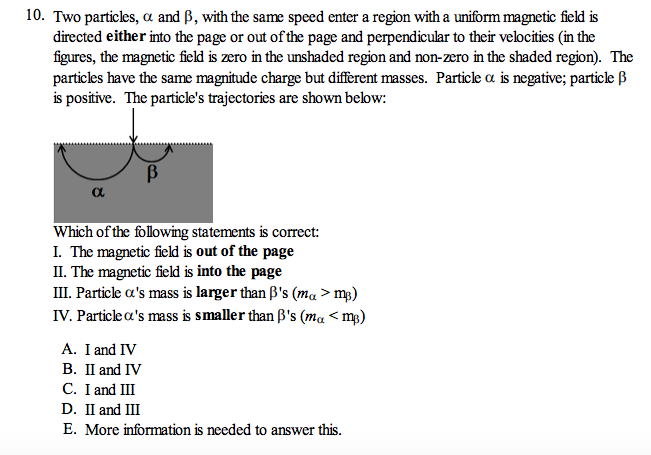Two particles, alpha and beta, with the same speed enter a region with a uniform magnetic field is directed either into the page or out of the page and perpendicular to their velocities (in the figures, the magnetic field is zero in the unshaded region and non-zero in the shaded region). The particles have the same magnitude charge but different masses. Particle alpha is negative; particle beta is positive. The particle's trajectories are shown below: Which of the following statements...

• ### 3. The diagram below shows the trajectories of three charged particles moving in a uniform magnetic...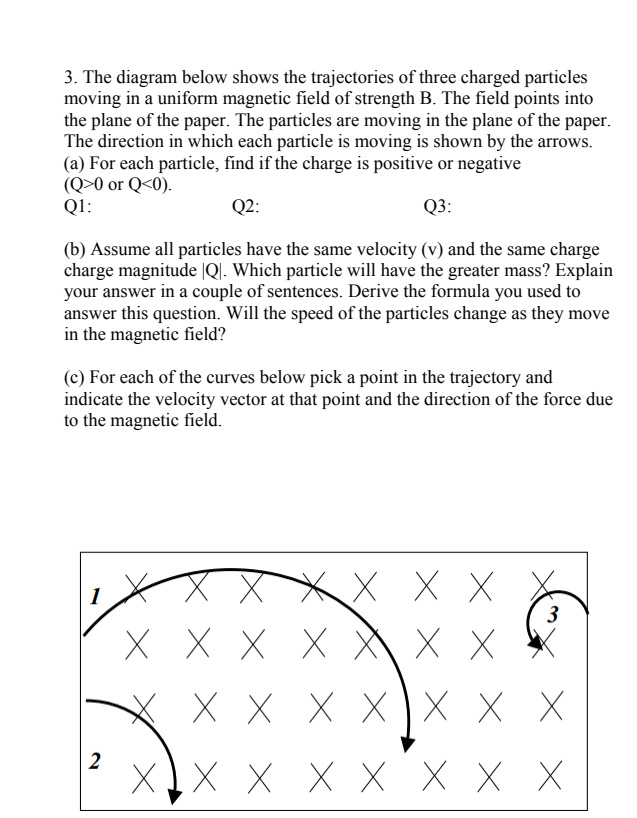3. The diagram below shows the trajectories of three charged particles moving in a uniform magnetic field of strength B. The field points into the plane of the paper. The particles are moving in the plane of the paper. The direction in which each particle is moving is shown by the arrows. (a) For each particle, find if the charge is positive or negative (Q>0 or Q<0). Q1: Q2: Q3: (b) Assume all particles have the same velocity (v) and...

• ### Learning Goal: To understand why charged particles move in circles perpendicular to a magnetic field and...

Learning Goal: To understand why charged particles move in circles perpendicular to a magnetic field and why the frequency is an invariant. A particle of charge q and mass m moves in a region of space where there is a uniform magnetic field B⃗ =B0k^(i.e., a magnetic field of magnitude B0 in the +z direction). (Figure 1) In this problem, neglect any forces on the particle other than the magnetic force. Part D If the resulting trajectory of the charged particle...

• ### A uniform magnetic field points out of the page. A charged particle is moving in the...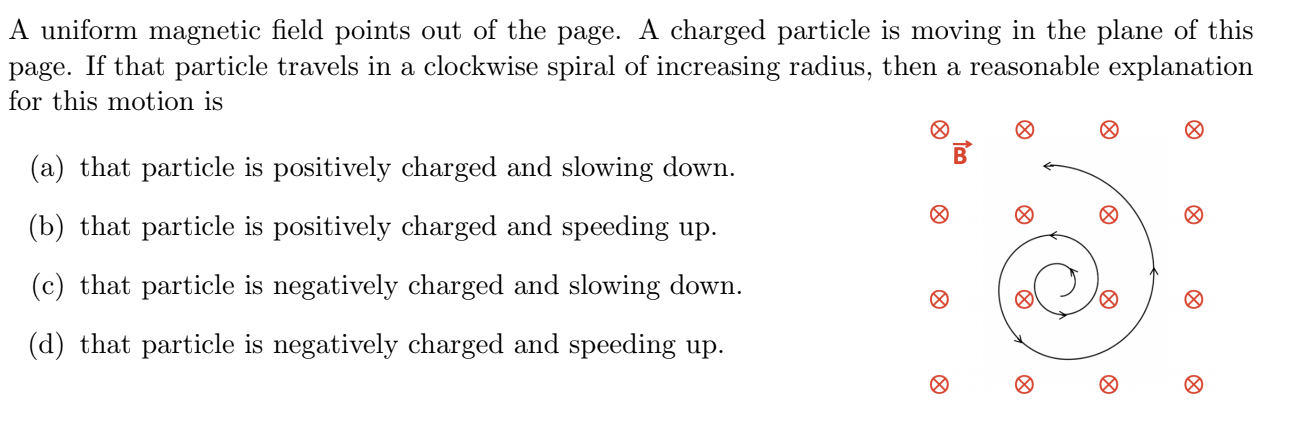A uniform magnetic field points out of the page. A charged particle is moving in the plane of this page. If that particle travels in a clockwise spiral of increasing radius, then a reasonable explanation for this motion is (a) that particle is positively charged and slowing down. (b) that particle is positively charged and speeding up. (c) that particle is negatively charged and slowing down. (d) that particle is negatively charged and speeding up.

• ### Two identical charged particles enter a uniform magnetic field. The velocity vector of each particle is...

Two identical charged particles enter a uniform magnetic field. The velocity vector of each particle is perpendicular to the magnetic field. If particle 2 has a speed that is twice that of particle 1, how do the periods of their circular motion compare (T1/T2)? a) 1 b) 1/2 c) 1 d) 2 e) 4

• ### Two identical charged particles enter a uniform magnetic field. The velocity vector of each particle is...

Two identical charged particles enter a uniform magnetic field. The velocity vector of each particle is perpendicular to the magnetic field. If particle 2 has a speed that is twice that of particle 1, how do the periods of their circular motion compare (T1/T2)?

Free Homework Help App